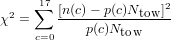## Ecological Detective: errata

October 14, 2011Last substantive change: 10 October 2001

These range from fussy typographical details (e.g., whether particular variables should be italicized) to fairly significant and/or confusing errors. Particularly important or confusing errors are marked *. See the ED web site http://www2.ucsc.edu/people/msmangel/ED.html for more details.

Thanks to Marc Mangel and Ray Hilborn for writing a great book that has relatively few errors, and to Tom Hobbs for pointing out several of the errors below.

Please contact me at bolker@mcmaster.ca if you know of any other errors in the text, or if you find mistakes in this list.

• p. 25 eq. 2.1, model B: uppercase A should be lowercase a for consistency
• p. 28 eq B2.1: first equals sign is spurious
• p. 38 para. 2 l. 2 read “model” for “mode”
• p. 53 second to last displayed equation, LHS should be Q(t + Δt) rather than Q(t + dt)
• p. 58 eq. 3.30: first m1 in equation should be italicized
• p. 58 eq. 3.31: last z (second line of equation, squared term) should be uppercase
• p. 61, ll. 2-3 after (3.41): uppercase A should be lowercase a
• pp. 66-68, (3.47-3.48, 3.50) and pseudocodes 3.1,3.3: all p before open parenthesis [p(] should be uppercase “P(” [or “Pr(” to match earlier notation)
• p. 68, (3.51): k in exponent should be italic
• *p. 73, first line of (3.65): sum should run from 1, not X
• p. 81, (3.84): a in denominator of last fraction should be italic
• p. 84, (3.91): s and r should be italic
• p. 90, pseudocode 3.4: σN doesn’t need to be specified (it’s a function of σW and σV ).
• *p. 103, (4.9) et seq.: The correct formula for the Pearson χ2 test should be in terms of numbers, not frequencies:(alternately, one can multiply the entire RHS of the formula given by Ntow). Furthermore, the discussion is slightly misleading: p(c) is rarely zero for most probability models, the problem is actually with expected counts < 5 (see any elementary statistics book for discussion of the Pearson test; one heuristic for this rule of thumb is that the Poisson distribution is reasonably close to Normal for expected means > 5).
• *p. 111: The parameter A ranges from -1 to 3.
• *p. 112, (5.5): in first eq., Aest should be A; in second eq., Best should be B; in third eq., Cest should be C
• p. 115, l. -7: replace “n parameters” by “mparameters” [ed: arguably, you could just change this sentence to “More generally, how do we compare models with different numbers of parameters?”]; just below eqn. 5.11, add “where n is the number of data points and m is the number of parameters”
• p. 126, l. 5 and -8 of main text, p. 129 l. 2: in each case the denominator of the fraction should have parentheses around it to be technically correct (and less confusing); so, SSQ*∕Nc - 6 SSQ*(Nc - 6), SSQ*∕Nc - 10 SSQ*(Nc - 10), and SSQ (single switch)∕Nc - 6 SSQ (single switch)(Nc - 6).
• p. 137, one line after eq. 7.12: “negative log-likelihood for 10 of the ten heights” should be “negative log-likelihood for n of the ten heights”
• p. 138, Figure 7.2: x axis label should be “Mean height (cm)” (not m)
• p. 154, l. 5 after eq. 7.42 “ratio of the logarithm” should be “logarithm of the ratio”
• *p. 156, first paragraph and pseudocode 7.4: the description of how Poisson variability is added disagrees with common sense and with Figure 7.7/Table 7.1. The book says:

Suppose that the number of individuals observed, Iobs, is the true number plus an observation uncertainty V that is Poisson distributed. Thus, Iobs = I + V will always equal or exceed the true number because V 0.

In Figure 7.7/Table 1.1, Iobs (black dots in figure; third column in table) is not the true number I (dashed line in figure; second column in table). Furthermore, following this interpretation makes it hard to figure out what the mean of the Poisson distributed term should be.

What is probably meant is that the observed value, Iobs, is drawn from a Poisson distribution with a mean of I. Following this interpretation:

• change “plus an observation uncertainty V that is Poisson distributed” on p. 156, ll. 5 ff. to “with an observation uncertainty that is Poisson distributed”;
• delete the next sentence, beginning “Thus, …”;
• step 4 in pseudocode 7.4 should read: “4. Calculate the actual observation by drawing a random number from a Poisson distribution whose mean is equal to the result from step 3.”.

The negative log-likelihood in Pseudocode 7.5, step 3c is Pr(I = Iobs|I ~ Pois(λ = Ipre))—the probability of drawing a sample Iobs from a Poisson distribution with mean Ipre. [ed: I haven’t checked this error with the authors, there is some possibility that I’m just confused—but I don’t think so]

• *p. 159, eqn 7.45: The definition of AIC should be Ai = 2L(Y |Mi*)+2pi where L(Y |Mi*) is the minimum value of the negative log-likelihood, evaluated at the best choice of parameters for model Mi